# Tag: Smullyan

Last time we had a brief encounter with the island of two truths, invented by Karin Cvetko-Vah. See her posts:

On this island, false statements have truth-value $0$ (as usual), but non-false statements are not necessarily true, but can be given either truth-value $Q$ (statements which the Queen on the island prefers) or $K$ (preferred by the King).

Think of the island as Trump’s paradise where nobody is ever able to say: “Look, alternative truths are not truths. They’re falsehoods.”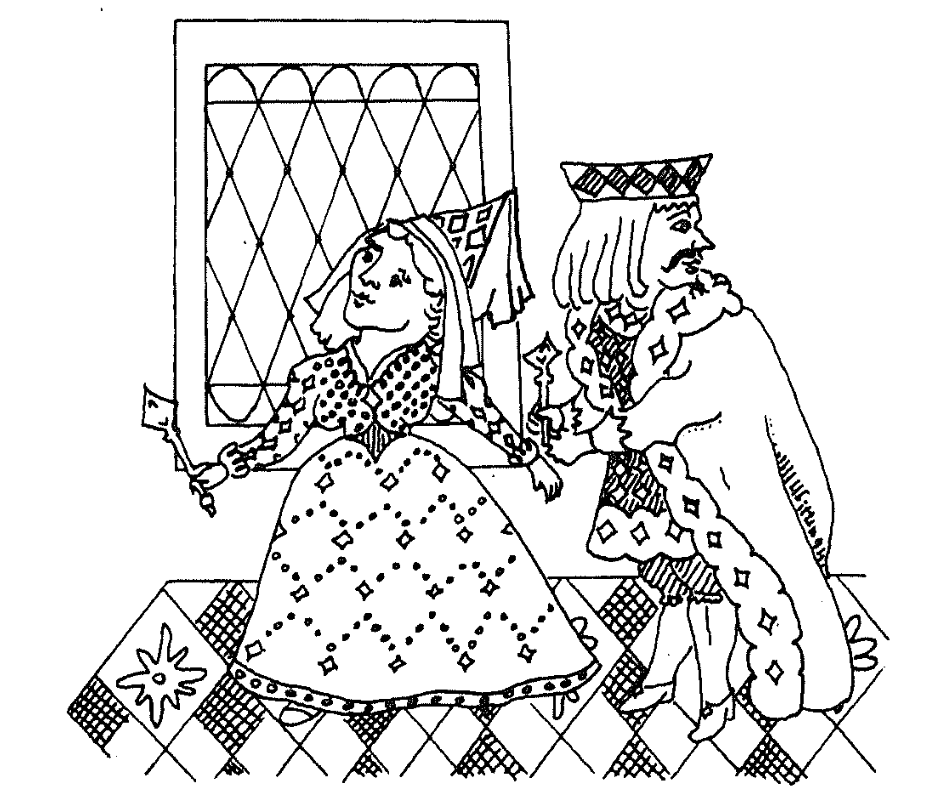Even the presence of just one ‘alternative truth’ has dramatic consequences on the rationality of your reasoning. If we know the truth-values of specific sentences, we can determine the truth-value of more complex sentences in which we use logical connectives such as $\vee$ (or), $\wedge$ (and), $\neg$ (not), and $\implies$ (then) via these truth tables:

$\begin{array}{c|ccc} \downarrow~\bf{\wedge}~\rightarrow & 0 & Q & K \\ \hline 0 & 0 & 0 & 0 \\ Q & 0 & Q & Q \\ K & 0 & K & K \end{array} \quad \begin{array}{c|ccc} \downarrow~\vee~\rightarrow & 0 & Q & K \\ \hline 0 & 0 & Q & K \\ Q & Q & Q & K \\ K & K & Q & K \end{array}$
$\begin{array}{c|ccc} \downarrow~\implies~\rightarrow & 0 & Q & K \\ \hline 0 & Q & Q & K \\ Q & 0 & Q & K \\ K & 0 & Q & K \end{array} \quad \begin{array}{c|c} \downarrow & \neg~\downarrow \\ \hline 0 & Q \\ Q & 0 \\ K & 0 \end{array}$

Note that the truth-values $Q$ and $K$ are not completely on equal footing as we have to make a choice which one of them will stand for $\neg 0$.

Common tautologies are no longer valid on this island. The best we can have are $Q$-tautologies (giving value $Q$ whatever the values of the components) or $K$-tautologies.

Here’s one $Q$-tautology (check!) : $(\neg p) \vee (\neg \neg p)$. Verify that $p \vee (\neg p)$ is neither a $Q$- nor a $K$-tautology.

Can you find any $K$-tautology at all?

Already this makes it incredibly difficult to adapt Smullyan-like Knights and Knaves puzzles to this skewed island. Last time I gave one easy example.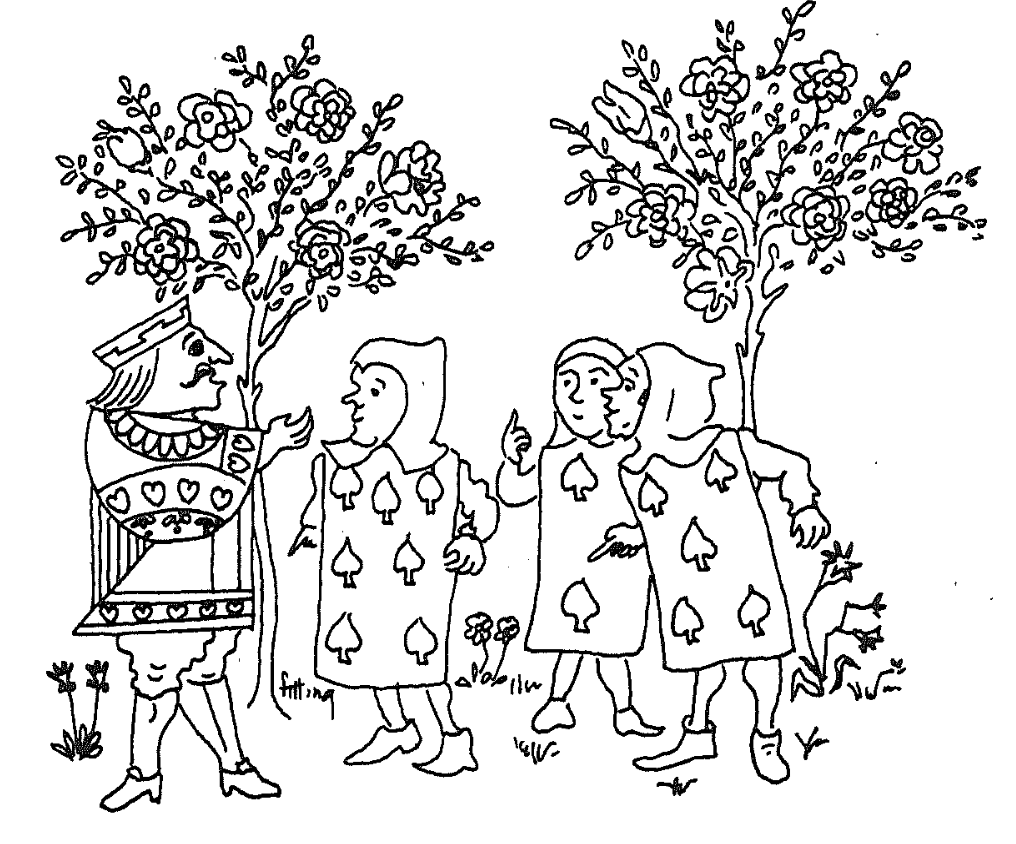Puzzle : On an island of two truths all inhabitants are either Knaves (saying only false statements), Q-Knights (saying only $Q$-valued statements) or K-Knights (who only say $K$-valued statements).

The King came across three inhabitants, whom we will call $A$, $B$ and $C$. He asked $A$: “Are you one of my Knights?” $A$ answered, but so indistinctly that the King could not understand what he said.

He then asked $B$: “What did he say?” $B$ replies: “He said that he is a Knave.” At this point, $C$ piped up and said: “That’s not true!”

Was $C$ a Knave, a Q-Knight or a K-Knight?

Solution : Q- and K-Knights can never claim to be a Knave. Neither can Knaves because they can only say false statements. So, no inhabitant on the island can ever claim to be a Knave. So, $B$ lies and is a Knave, so his stament has truth-value $0$. $C$ claims the negation of what $B$ says so the truth-value of his statement is $\neg 0 = Q$. $C$ must be a Q-Knight.

As if this were not difficult enough, Karin likes to complicate things by letting the Queen and King assign their own truth-values to all sentences, which may coincide with their actual truth-value or not.

Clearly, these two truth-assignments follow the logic of the island of two truths for composed sentences, and we impose one additional rule: if the Queen assigns value $0$ to a statement, then so does the King, and vice versa.

I guess she wanted to set the stage for variations to the island of two truths of epistemic modal logical puzzles as in Smullyan’s book Forever Undecided (for a quick summary, have a look at Smullyan’s paper Logicians who reason about themselves).

A possible interpretation of the Queen’s truth-assignment is that she assigns value $Q$ to all statements she believes to be true, value $0$ to all statements she believes to be false, and value $K$ to all statements she has no fixed opinion on (she neither believes them to be true nor false). The King assigns value $K$ to all statements he believes to be true, $0$ to those he believes to be false, and $Q$ to those he has no fixed opinion on.

For example, if the Queen has no fixed opinion on $p$ (so she assigns value $K$ to it), then the King can either believe $p$ (if he also assigns value $K$ to it) or can have no fixed opinion on $p$ (if he assigns value $Q$ to it), but he can never believe $p$ to be false.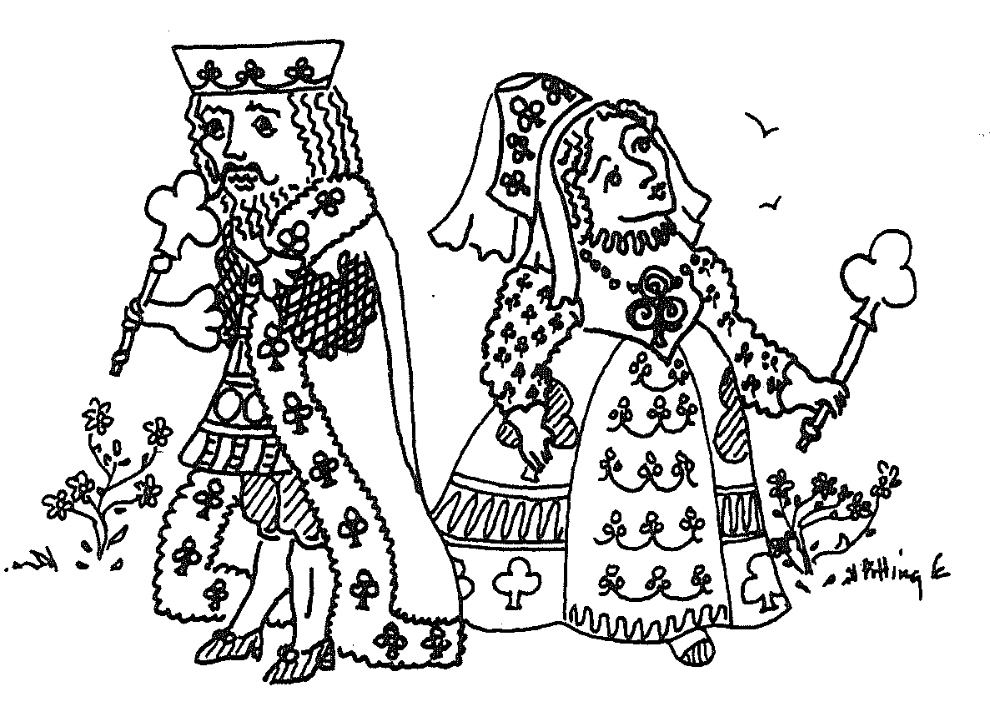Puzzle : We say that Queen and King ‘agree’ on a statement $p$ if they both assign the same value to it. So, they agree on all statements one of them (and hence both) believe to be false. But there’s more:

• Show that Queen and King agree on the negation of all statements one of them believes to be false.
• Show that the King never believes the negation of whatever statement.
• Show that the Queen believes all negations of statements the King believes to be false.

Solution : If one of them believes $p$ to be false (s)he will assign value $0$ to $p$ (and so does the other), but then they both have to assign value $Q$ to $\neg p$, so they agree on this.

The value of $\neg p$ can never be $K$, so the King does not believe $\neg p$.

If the King believes $p$ to be false he assigns value $0$ to it, and so does the Queen, but then the value of $\neg p$ is $Q$ and so the Queen believes $\neg p$.

We see that the Queen and King agree on a lot of statements, they agree on all statements one of them believes to be false, and they agree on the negation of such statements!

Can you find any statement at all on which they do not agree?

Well, that may be a little bit premature. We didn’t say which sentences about the island are allowed, and what the connection (if any) is between the Queen and King’s value-assignments and the actual truth values.

For example, the Queen and King may agree on a classical ($0$ or $1$) truth-assignments to the atomic sentences for the island, and replace all $1$’s with $Q$. This will give a consistent assignment of truth-values, compatible with the island’s strange logic. (We cannot do the same trick replacing $1$’s by $K$ because $\neg 0 = Q$).

Clearly, such a system may have no relation at all with the intended meaning of these sentences on the island (the actual truth-values).

That’s why Karin Cvetko-Vah introduced the notions of ‘loyalty’ and ‘sanity’ for inhabitants of the island. That’s for next time, and perhaps then you’ll be able to answer the question whether Queen and King agree on all statements.

(all images in this post are from Smullyan’s book Alice in Puzzle-Land)Raymond Smullyan‘s logic puzzles are legendary. Among his best known are his Knights (who always tell the truth) and Knaves (who always lie) puzzles. Here’s a classic example.

“On the day of his arrival, the anthropologist Edgar Abercrombie came across three inhabitants, whom we will call $A$, $B$ and $C$. He asked $A$: “Are you a Knight or a Knave?” $A$ answered, but so indistinctly that Abercrombie could not understand what he said.

He then asked $B$: “What did he say?” $B$ replies: “He said that he is a knave.” At this point, $C$ piped up and said: “Don’t believe that; it’s a lie!”

Was $C$ a Knight or a Knave?”

If you are stumped by this, try to figure out what kind of inhabitant can say “I am a Knave”.

Some years ago, my friend and co-author Karin Cvetko-Vah wrote about a much stranger island, the island of two truths.

“The island was ruled by a queen and a king. It is important to stress that the queen was neither inferior nor superior to the king. Rather than as a married couple one should think of the queen and the king as two parallel powers, somewhat like the Queen of the Night and the King Sarastro in Mozart’s famous opera The Magic Flute. The queen and the king had their own castle each, each of them had their own court, their own advisers and servants, and most importantly each of them even had their own truth value.

On the island, a proposition p is either FALSE, Q-TRUE or K-TRUE; in each of the cases we say that p has value 0, Q or K, respectively. The queen finds the truth value Q to be superior, while the king values the most the value K. The queen and the king have their opinions on all issues, while other residents typically have their opinions on some issues but not all.”

The logic of the island of two truths is the easiest example of what Karin and I called a non-commutative frame or skew Heyting algebra (see here), a notion we then used, jointly with Jens Hemelaer, to define the notion of a non-commutative topos.If you take our general definitions, and take Q as the distinguished top-element, then the truth tables for the island of two truths are these ones (value of first term on the left, that of the second on top):

$\begin{array}{c|ccc} \wedge & 0 & Q & K \\ \hline 0 & 0 & 0 & 0 \\ Q & 0 & Q & Q \\ K & 0 & K & K \end{array} \quad \begin{array}{c|ccc} \vee & 0 & Q & K \\ \hline 0 & 0 & Q & K \\ Q & Q & Q & K \\ K & K & Q & K \end{array} \quad \begin{array}{c|ccc} \rightarrow & 0 & Q & K \\ \hline 0 & Q & Q & K \\ Q & 0 & Q & K \\ K & 0 & Q & K \end{array} \quad \begin{array}{c|c} & \neg \\ \hline 0 & Q \\ Q & 0 \\ K & 0 \end{array}$

Note that on this island the order of statements is important! That is, the truth value of $p \wedge q$ may differ from that of $q \wedge p$ (and similarly for $\vee$).

Let’s reconsider Smullyan’s puzzle at the beginning of this post, but now on an island of two truths, where every inhabitant is either of Knave, or a Q-Knight (uttering only Q-valued statements), or a K-Knight (saying only K-valued statements).

Again, can you determine what type $C$ is?

Well, if you forget about the distinction between Q- and K-valued sentences, then we’re back to classical logic (or more generally, if you divide out Green’s equivalence relation from any skew Heyting algebra you obtain an ordinary Heyting algebra), and we have seen that then $B$ must be a Knave and $C$ a Knight, so in our new setting we know that $C$ is either a Q-Knight or a K-Knight, but which of the two?

Now, $C$ claims the negation of what $B$ said, so the truth value is $\neg 0 = Q$, and therefore $C$ must be a Q-Knight.

Recall that in Karin Cvetko-Vah‘s island of two truths all sentences have a unique value which can be either $0$ (false) or one of the non-false values Q or K, and the value of combined statements is given by the truth tables above. The Queen and King both have an opinion on all statements, which may or may not coincide with the actual value of that statement. However, if the Queen assigns value $0$ to a statement, then so does the King, and conversely.

Other inhabitants of the island have only their opinion about a subset of all statements (which may be empty). Two inhabitants agree on a statement if they both have an opinion on it and assign the same value to it.

Now, each inhabitant is either loyal to the Queen or to the King (or both), meaning that they agree with the Queen (resp. King) on all statements they have an opinion of. An inhabitant loyal to the Queen is said to believe a sentence when she assigns value $Q$ to it (and symmetric for those loyal to the King), and knows the statement if she believes it and that value coincides with the actual value of that statement.

Further, if A is loyal to the Queen, then the value of the statement ‘A is loyal to the Queen’ is Q, and if A is not loyal to the Queen, then the value of the sentence ‘A is loyal to the Queen’ is $0$ (and similarly for statements about loyalty to the King).

These notions are enough for the first batch of ten puzzles in Karin’s posts

Just one example:

Show that if anybody on the island knows that A is not loyal to the Queen, then everybody that has an opinion about the sentence ‘A is loyal to the Queen’ knows that.

After these two posts, Karin decided that it was more fun to blog about the use of non-commutative frames in data analysis.

But, she once gave me a text containing many more puzzles (as well as all the answers), so perhaps I’ll share these in a follow-up post.

Raymond Smullyan‘s logic puzzles frequently involve Knights (who always tell the truth) and Knaves (who always lie).

In his book Logical Labyrinths (really a first course in propositional logic) he introduced islands where the lying or truth-telling habits can vary from day to day—that is, an inhabitant might lie on some days and tell the truth on other days, but on any given day, he or she lies the entire day or tells the truth the entire day.

An island is said to be Boolean if is satisfies the following conditions:

• $\mathbf{N}$ : For any inhabitant $A$ there is an inhabitant who tells the truth on all and only those days on which $A$ lies.
• $\mathbf{M}$ : For any inhabitants $A$ and $B$ there is an inhabitant $C$ who tells the truth on all and only those days on which $A$ and $B$ both tell the truth.
• $\mathbf{J}$ : For any inhabitants $A$ and $B$ there is an inhabitant $C$ who tells the truth on all and only those days on which either $A$ tells the truth or $B$ tells the truth (or both). (In other words, $C$ lies on those and only those days on which $A$ and $B$ both lie.)

On any given day there are only Knights and Knaves on the island, but these two populations may vary from one day to the other. The subsets (of all days) for which there is an inhabitant who is a Knight then and a Knave on all other days form a Boolean algebra with operations $\wedge = \cap$ ($\mathbf{M}$eet), $\vee= \cup$ ($\mathbf{J}$oin) and $\neg=$ set-complement ($\mathbf{N}$egation).

Here’s a nice puzzle from Smullyan’s book:

Solomon’s Island also turned out to be quite interesting. When Craig arrived on it, he had the following conversation with the resident sociologist:

Craig : Is this island a Boolean island?
Sociologist : No.
Craig : Can you tell me something about the lying and truth-telling habits of the residents here?
Sociologist : For any inhabitants $A$ and $B$, there is an inhabitant $C$ who tells the truth on all and only those days on which either $A$ lies or $B$ lies (or both).

Show that the sociologist didn’t go native, and that his research is lousy.
(My wording, not Smullyan’s)

Smullyan’s version: This interview puzzled inspector Craig; he felt that something was wrong. After a while he realized for sure that something was wrong, the sociologist was either lying or mistaken!

Extending Smullyan’s idea, we can say that an island is Heyting if, in addition to $\mathbf{M}$ and $\mathbf{J}$ is satisfies the following rules

• $\mathbf{T}$ : at least one inhabitant tells the truth on all days.
• $\mathbf{F}$ : at least one inhabitant lies on all days.
• $\mathbf{I}$ : For any inhabitants $A$ and $B$ there is an inhabitant $C$ sharing Knight-days with $A$ only when $B$ tells the truth, and there are no inhabitants doing this while telling the truth on more days than $C$.

Let’s give an example of an Heyting island which is not Boolean.

On Three-island there are only three kinds of people: Knights, Knaves and Alternates, who can neither lie nor tell the truth two days in a row. All Alternates tell the truth on the same days.

Here’s a riddle:

You meet John, who is a Knight, James, an Alternate, and William, a Knave. You don’t know who is who. You can only ask one question containing at most four words, giving you a Yes or No answer, to just one of the three. The answer must tell you whether that person is James or not.

You may like to watch Smullyan on the Carson show for a hint.

Or, you might just watch it reminiscing long forgotten times, when talkshow-hosts still listened to their guests, and could think for themselves…

“A subway is just a hole in the ground, and that hole is a maze.”

“The map is the last vestige of the old system. If you can’t read the map, you can’t use the subway.”

Eddie Jabbour in Can he get there from here? (NYT)

Sometimes, lines between adjacent stations can be uni-directional (as in the Paris Metro map below in the right upper corner, 7bis). So, it is best to view a subway map as a directed graph, with vertices the different stations, and directed arrows when there’s a service connecting two adjacent stations.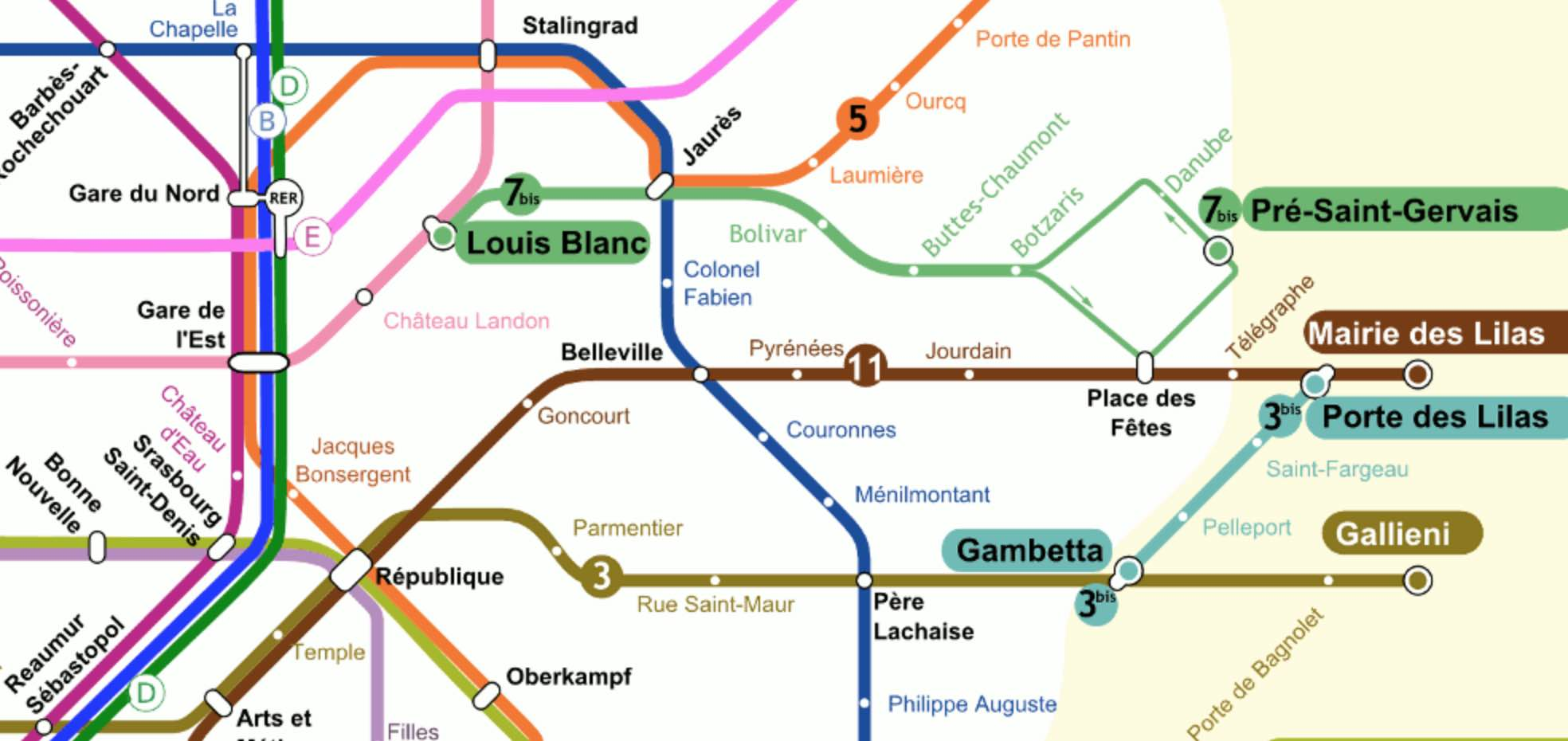Aha! But, directed graphs form a presheaf topos. So, each and every every subway in the world comes with its own logic, its own bi-Heyting algebra!

Come again…?

Let’s say Wally (or Waldo, or Charlie) is somewhere in the Paris metro, and we want to find him. One can make statements like:

$P$ = “Wally is on line 3bis from Gambetta to Porte des Lilas.”, or

$Q$ = “Wally is traveling along line 11.”

Each sentence pinpoints Wally’s location to some directed subgraph of the full Paris metro digraph, let’s call this subgraph the ‘scope’ of the sentence.

We can connect such sentences with logical connectives $\vee$ or $\wedge$ and the scope will then be the union or intersection of the respective scopes.

The scope of $P \vee Q$ is the directed subgraph of line 11 (in both directions) together with the directed subgraph of line 3bis from Gambetta to Porte des Lilas.

The scope of $P \wedge Q$ is just the vertex corresponding to Porte des Lilas.

The scope of the negation $\neg R$ of a sentence $R$ is the subgraph complement of the scope of $R$, so it is the full metro graph minus all vertices and directed edges in $R$-scope, together with all directed edges starting or ending in one of the deleted vertices.

For example, the scope of $\neg P$ does not contain directed edges along 3bis in the reverse direction, nor the edges connecting Gambetta to Pere Lachaise, and so on.

In the Paris metro logic the law of double negation does not hold.$\neg \neg P \not= P$ as both statements have different scopes. For example, the reverse direction of line 3bis is part of the scope of $\neg \neg P$, but not of $P$.

So, although the scope of $P \wedge \neg P$ is empty, that of $P \vee \neg P$ is not the full digraph.

The logical operations $\vee$, $\wedge$ and $\neg$ do not turn the partially ordered set of all directed subgraphs of the Paris metro into a Boolean algebra structure, but rather a Heyting algebra.

Perhaps we were too drastic in removing all “problematic edges” from the scope of $\neg R$ (those with a source or target station belonging to the scope of $R$)?

We might have kept all problematic edges, and added the missing source and/or target stations to get the scope of another negation of $R$: $\sim R$.

Whereas the scope of $\neg \neg R$ always contains that of $R$, the scope of $\sim \sim R$ is contained in $R$’s scope.

The scope of $R \vee \sim R$ will indeed be the whole graph, but now $R \wedge \sim R$ does no longer have to be empty. For example, $P \wedge \sim P$ has as its scope all stations on line 3bis.

In general $R \wedge \sim R$ will be called the ‘boundary’ $\partial(R)$ of $R$. It consists of all stations within $R$’s scope that are connected to the outside of $R$’s scope.

The logical operations $\vee$, $\wedge$, $\neg$ and $\sim$ make the partially ordered set of all directed subgraphs of the Paris metro into a bi-Heyting algebra.

There’s plenty more to say about all of this (and I may come back to it later). For the impatient, there’s the paper by Reyes and Zolfaghari: Bi-Heyting Algebras, Toposes and Modalities.

Right now, I’m more into exploring whether this setting can be used to revive an old project of mine: Heyting Smullyanesque problems (btw. the algebra in that post is not Heyting, oops!).

Raymond Smullyan brought Knights and Knaves puzzles to a high art in his books. Here’s the setting:

On Smullyan’s islands there are Knights, who always tell true statements, Knaves, who always lie, and sometimes also Normals, who sometimes tell the truth and sometimes lie.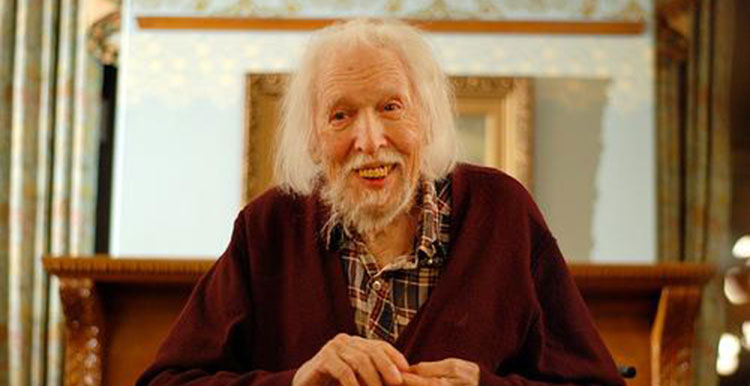(image credit MikeKlein)

Problems of this sort can be solved by classical propositional logic, replacing every sentence $P$ told by inhabitant $A$ by the formula $k_A \leftrightarrow P$ where $k_A$ is the sentence “A is a Knight”.

Some time ago I asked for Smullyanesque problems in a non-classical logic, where we replace the usual truth-values $T$ (true) and $F$ (false) of propositional logic by elements of an Heyting algebra.

Jason Rosenhouse wrote a paper Knights, Knaves, Normals, and Neutrals for The College Mathematics Journal, containing more information than in his blog post, as well as some nice challenging puzzles. Here’s Rosenhouse’s setting:

Apart from Knights, Knaves and Normals there’s also the tribe of Neutrals (which he describes as a sort of trans-Knights or trans-Knaves) who can only tell sentences with truth value $N$ in the Heyting algebra $\{ T,N,F \}$ with logical connectives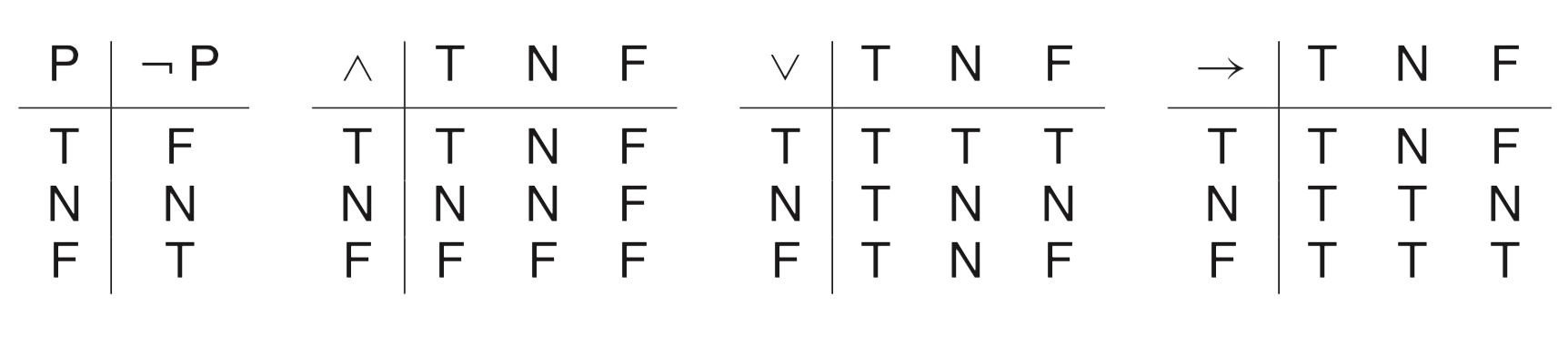A typical sentence of truth value $N$ is “A is a Knight” or “A is a Knave” whereas, in reality, $A$ is Neutral. Here are the truth values of sentences about a person (the column headings) with respect to the actual situation (the row headings)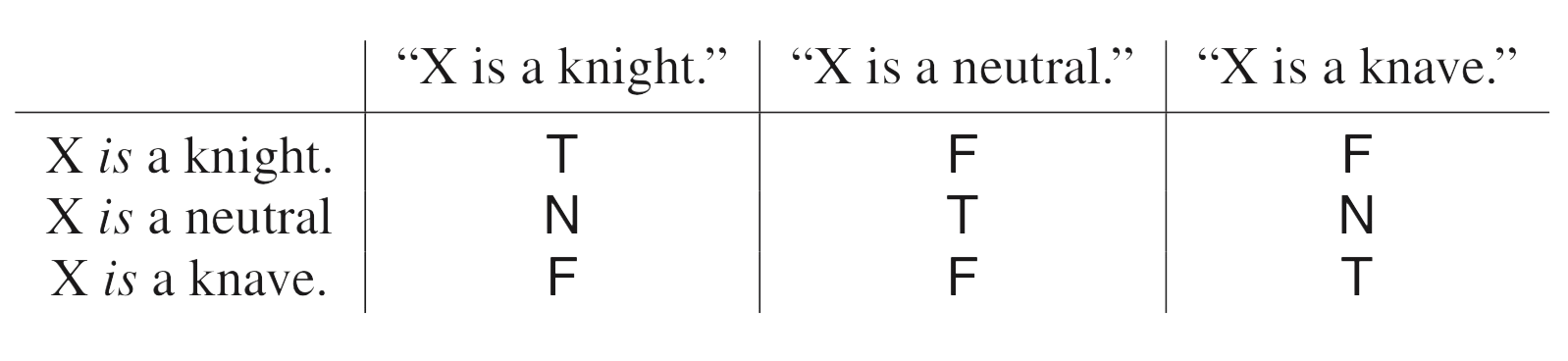A good way into such problems is to focus on sentence like “A is a Neutral” as they have only classical truth values $T$ or $F$ and to remember that Neutrals can never say a sentence with classical truth value. Here’s an example (only Knights,Knaves or Neutrals present):

Problem 1: Suppose you meet three people, named Dave, Evan and Ford. They make the following statements:

Dave: Evan is a knight.
Evan: Ford is a knave.
Ford: Dave is a neutral.

Can you determine the types of all three people?

Solution: Ford’s sentence has value $T$ or $F$, so Ford cannot be Neutral so must be a Knight or Knave. But then Evan’s sentence also has classical truth value, so he can’t be Neutral either, and by the same reasoning also Dave cannot be neutral. So, we know that all three people must be either Knights or Knaves and then it follows at once that Ford is a Knave (because he lied) and that Evan and Dave must be knights.

These puzzles become more interesting once we use logical connectives.

Problem 2: What can you conclude from this dialog?

Mimi: Olaf is a Knight and Olaf is not a Knight.
Nate: Olaf is a Knave or Olaf is not a Knave.
Olaf: Mimi is a Knight or Nate is a Knave or I am Neutral.

Solution: Mimi’s sentence can only have truth value $F$ or $N$ so she can’t be a Knight. Nate’s sentence has value $T$ or $N$ so he cannot be a Knave.
The two first parts of Olaf’s line cannot be $T$ so must be either $N$ or $F$. So, Olav’s line has total value $T$ only if the last part ‘I am Neutral’ is $T$. So, Olaf can neither be a Knight (because then the last part is $F$) nor Neutral (because then the line would have value $T$ and Neutrals can only speak $N$-sentences). So, Olaf must be a knave. Nate must then be a Knight and Mimi a Knave (because the first part of her line is $F$ and so must be the whole sentence).

The situation becomes even more complicated when we allow Normals (who sometimes lie and sometimes tell the truth but never say sentences with value $N$) to be present. Here’s Rosenhouse’s ‘Grand Finale’:

Problem 3: One day a visitor encountered eight people, among them exactly two Knights, two Knaves, two Normals and two Neutrals. What can you conclude from this dialog:

Sara: Walt is a Neutral or Vera is a Neutral.
Todd: Xara is a Knave and Yoav is a Knave.
Ursa: If Sara is a Knight, then Todd and Yoav are Normals.
Vera: I am not a Neutral.
Walt: If Vera is not a Neutral, then neither is Todd.
Xara: Todd is a Knight if and only if Zack is a Neutral.
Zack: Xara is a Neutral if and only if Walt is not a Normal.

You will solve this problem much quicker than to read through the long explanation in Rosenhouse’s paper.

These puzzles beg to be generalised to more complicated Heyting algebras.

What about a book on “Knights, Knaves and Knols”? A Knol (or $X$-Knol) has knowledge limited to sentences with truth value $X$, an element in the Heyting algebra.(image credit: Joe Blitzstein via Twitter)

Smullyan’s Knights and Knaves problems are classics. On an island all inhabitants are either Knights (who only tell true things) and Knaves (who always lie). You have to determine their nature from a few statements. Here’s a very simple problem:

“Abercrombie met just two inhabitants, A and B. A made the following statement: “Both of us are Knaves.” What is A and what is B?”

Now, this one is simple enough to solve, but for more complicated problems a generic way to solve the puzzles is to use propositional calculas, as explained in Smullyan’s Logical Labyrinths”, chapter 8 “Liars, truth-tellers and propositional logic’.

If an inhabitants $A$ asserts a proposition $P$, and if $k_A$ is the assertion ‘$A$ is a Knight’, then the statement can be rephrased as

$k_A \Leftrightarrow P$

for if $A$ is a Knight, $P$ must be true and if $A$ is a Knave $P$ must be false.

Usually, one can express $P$ as a propositional statement involving $k_A,k_B,k_C,\dots$.
The example above can be rephrased as

$k_A \Leftrightarrow (\neg k_A \wedge \neg k_B)$

Assigning truth values to $k_A$ and $k_B$ and setting up the truth-table for this sentence, one sees that the only possibility for this to be true is that $k_A$ equals $0$ and $k_B$ equals $1$. So, $A$ is a Knave and $B$ is a Knight.

Clearly, one only requires this approach for far more difficult problems.

In almost all Smullyan puzzles, the only truth values are $0$ and $1$. There’s a short excursion to Boolean algebras (sorry, Boolean islands) in chapter 9 ‘Variable Liars’ in Logical Labyrinths. But then, the type of problems are about finding equivalent notions of Boolean algebras, rather that generalised Knights&Knaves puzzles.

Did anyone pursue the idea of Smullyanesque puzzles with truth values in a proper Heyting algebra?

I only found one blog-post on this: Non-Classical Knights and Knaves by Jason Rosenhouse.

He considers three valued logic (the Heyting algebra corresponding to the poset 0-N-1, and logical connectives as in the example on the Wiki-page on Heyting algebras.

On his island the natives cycle, repeatedly and unpredictably, between the two states. They are knights for a while, then they enter a transitional phase during which they are partly knight and partly knave, and then they emerge on the other side as knaves.

“If Joe is in the transitional phase, and you say, “Joe is a knight,” or “Joe is a knave,” what truth value should we assign to your statement? Since Joe is partly knight and partly knave, neither of the classical truth values seems appropriate. So we shall assign a third truth value, “N” to such statements. Think of N as standing for “neutral” or “neither true nor false.” On the island, vague statements are assigned the truth value N.

Just to be clear, it’s not just any statement that can be assigned the truth value N. It is only vague statements that receive that truth value, and for now our only examples of such statements are attributions of knight-hood and knave-hood to people in the transitional phase.

For the natives, entering the transitional phase implied a disconcerting loss of identity. Uncertain of how to behave, they hedged their bets by only making statements with truth value N. People in the transitional phase were referred to as neutrals. So there are now three kinds of people: Knights, who only make true statements; Knaves, who only make false statements; and Neutrals, who only make statements with the truth value N.”

He gives one example of a possible problem:

“Suppose you meet three people, named Dave, Evan and Ford. They make the following statements:

Dave: Evan is a knight.
Evan: Ford is a knave.
Ford: Dave is a neutral.

Can you determine the types of all three people?”Smullyan found himself in a very strange country indeed!

All the inhabitants of this country are completely truthful – they always tell you honestly what they believe, but the trouble is that about half of the population are totally mad, and all their beliefs are wrong!

The other half are totally sane and accurate in their judgments, all their beliefs are correct.

Smullyan felt honoured to be invited by the Presidential couple.

The President was the only one who said anything, and what he said was:

“My wife once said that I believe that she believes I am mad.”

What can be deduced about the President’s sanity, and that of his wife?

Reference: This is a minor adaptation of Problem 4.5 in Raymond Smullyan‘s book Logical Labyrinths.